### Creating simple equations

You can perform simple mathematical operations such as 1+1 directly in a cell. Quattro Pro interprets these equations as formulas and indicates cells with formulas by adding a blue triangle to the bottom-left corner of the cell. Quattro Pro also totals values for you.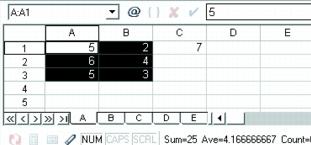In this example, the sum of the selected cells (columns A and B, rows 1 through 3) is displayed on the application bar.

You can also specify to have Quattro Pro identify the forward slash ( / ) as a division sign.

For example, when the forward slash is used as a division sign, 4/4 is interpreted as 4 divided by 4, and the result 1 displays. Otherwise, when you type a forward slash, Quattro Pro might assume you are typing a date. For example, 4/4 might be interpreted as the date 04/04.

#### To do simple math in cells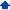1 Type the numbers and math operators in a cell.
 For example, type 4500+450.
 2 Press Enter.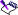• Do not include commas in numbers.

#### To total values in a column1 Click in the first blank cell below and one column to the left of the cells to be totaled.
 2 Type total.
 3 Press Enter.• This feature is not case sensitive and is language dependent.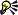• You can also total values in a row by clicking the first blank cell to the right and one row above the cells to be totaled, and typing total. Press Enter.

#### To set the forward slash (/) as a division sign1 Click Tools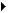Settings.
 2 In the list of categories, double-click Workspaces, Application, and click General.
 3 Enable the Mathematical formula entry check box.• You can also identify the forward slash as a division sign by typing a plus sign (+) before the equation (for example, +4/12).